Courses

# Test: Colligative Properties - 2

## 30 Questions MCQ Test Physical Chemistry | Test: Colligative Properties - 2

Description
This mock test of Test: Colligative Properties - 2 for Chemistry helps you for every Chemistry entrance exam. This contains 30 Multiple Choice Questions for Chemistry Test: Colligative Properties - 2 (mcq) to study with solutions a complete question bank. The solved questions answers in this Test: Colligative Properties - 2 quiz give you a good mix of easy questions and tough questions. Chemistry students definitely take this Test: Colligative Properties - 2 exercise for a better result in the exam. You can find other Test: Colligative Properties - 2 extra questions, long questions & short questions for Chemistry on EduRev as well by searching above.
QUESTION: 1

Solution:
QUESTION: 2

Solution:
QUESTION: 3

### Select correct statement?

Solution:
QUESTION: 4

The boiling point of an azeotropics mixture of water–ethanol is less than that of both water and ethanol. Then:

Solution:
QUESTION: 5

Formation of a solut ion from two components can be considered as:

(i) Pure solvent → separated solvent molecules, ΔH1
(ii) Pure solute → separated solute mo lecules, ΔH2
(iii) Separated solvent and solute molecules → solution, ΔH3

Solution so formed will be ideal if:

Solution:
QUESTION: 6

Total vapour pressure of mixture of 1 mol X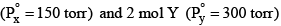is 240 torr. In this case:

Solution:
QUESTION: 7

In mixture of A and B, components show positive deviation when:

Solution:
QUESTION: 8

Which will form maximum boiling azeotrope:

Solution:
QUESTION: 9

Total vapour pressure of mixture of 1 mole of volatile component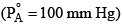and 3 mole of volatile component B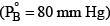is 90 mm Hg. For such case:

Solution: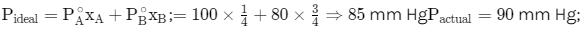∵ Actual v. pr. is greater than ideal solution v.pr. so +ve deviation from Raoult's law

QUESTION: 10

The azeotropics mixture of water (B.P. = 100°C) and HCl (B.P. = 86°C) boils at about 120°C. During fractional distillation of this mixture it is possible to obtain:

Solution:
QUESTION: 11

An azeotropic mixture of two liquids has a boiling point higher than either of them when it:

Solution:
QUESTION: 12

If two liquids A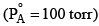and B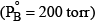are completely immiscible wit h each other (each one will behave independently of the other) are present in a closed vessel. The total vapour pressure of the system will be:

Solution:
QUESTION: 13

When a liquid that is immiscible with water was steam distilled at 95.2°C at a total pressure of 748 torr, the distillate contained 1.25 g of the liquid per gram of water. The vapour pressure of water is 648 torr at 95.2°C, what is the molar mass of liquid?

Solution:
QUESTION: 14

Water and Chlorobenzene are immiscible liquids. Their mixture boils at 89°C under a reduced pressure of 7.7×104 Pa. The vapour pressure of pure water at 89°C is 7×104 Pa. Weight per cent of Chlorobenzene in the distillate is:

Solution:
QUESTION: 15

Which of the following is not a Colligative property?

Solution:
QUESTION: 16

The degree of dissociation of an electrolyte is a α and its van’t Hoff factor is i. The number of ions obtained by complete dissociation of 1 molecule of the electrolyte is:

Solution:
QUESTION: 17

The van’t Hoff factor I for an electrolyte which undergoes dissociation and association in solvent are respectively:

Solution:
QUESTION: 18

Which solution has the highest vapour pressure?

Solution:
QUESTION: 19

An aqueous solution is 1.00 molal in KI. Which change will cause the vapour pressure of the solution to increase:

Solution:
QUESTION: 20

Four solutions of K2SO4 with the concentrations 0.1 m, 0.01 m, 0.001 m and 0.0001 m are available. The maximum value of Colligative property corresponds to:

Solution:
QUESTION: 21

Moles of Na2SO4 to be dissolved in 12 mole water to lower its vapour pressure by 10 mm Hg at a temperature at which vapour pressure of pure water is 50 mm is:

Solution:
QUESTION: 22

A very diluted saturated solution of a sparingly soluble salt X3Y4 has a vapour pressure of 20 mm Hg at temperature T, while pure water exerts a pressure of 20.0126 mm Hg at the same temperature. Calculate molality (m) at temperature T:

Solution:
QUESTION: 23

When 1 mole of a solute is dissolved in 1 kg of H2O, boiling point of solution was found to be 100.5°C. Kb for H2O is:

Solution:
QUESTION: 24

Chloro form, CHCl3, boils at 61.7°C. If the Kb for chloroform is 3.63°C/molal, what is the boiling point of a solution of 15.0 kg of CHCl3 and 0.616 kg of acenaphthalene, C12H10?

Solution:
QUESTION: 25

A compound has the empirical formula C10H8Fe. A solution of 0.26 g of the compound in 11.2 g of benzene (C6H6) boils at 80.26°C. The boiling point of benzene is 80.10°C; the Kb is 2.53°C/molal. What is the molecular formula of the compound?

Solution:
QUESTION: 26

A solution of 0.640 g of azulene in 100.0 g of benzene bo ils at 80.23°C. The boiling point of benzene is 80.10°C; the Kb is 2.53°C/molal. What is the molecular weight of azulene?

Solution:
QUESTION: 27

One molal solution of a carboxylic acid in benzene shows the elevation of boiling point of 1.518 K. The degree of association for dimerization of the acid in benzene is (Kb for benzene = 2.53 K kg mol–1):

Solution:
QUESTION: 28

The boiling point elevation constant for toluene is 3.32 K kg mol–1. The normal boiling point of toluene is 110.7°C. The enthalpy of vaporization of toluene would be nearly:

Solution:
QUESTION: 29

Which one of the following aqueous solutions will exhibit highest boiling point:

Solution:
QUESTION: 30

When a solution containing non–volatile solute freezes, which equilibrium would exist ?

Solution: